## How Much Force Applied to a Rig Tong To Get The Right Torque at The ConnectionOne application of the rig tong is to use to make up connection. The question is asked about how to get the right torque value to the connection if you use the rig tong because you will not see the torque value on the gauge.This article will describe how to determine the correct force applied to get the correct torque value when you use the rig tong to make up the connection.

## Rig Derrick Load Calculation

The drilling line is reeved over a set of crow block and down to another set of sheaves known as travelling block. The hook connected to travelling block is used to suspend the drilling load. One end of drilling line is wound onto the drawworks and this line is called “Fast Line”. Other end of the drilling line is tied into an anchor point on the rig floor and this line is named as “Dead Line”. The drilling line is reeved around the blocks several times in order to meet required load.According to the diagram above, we can use basic physic to determine derrick load.

## Drillstring Elongation Due To a Tensile Load

When a tensile load is applied into a drillstring, it results in elongation of the drillstring.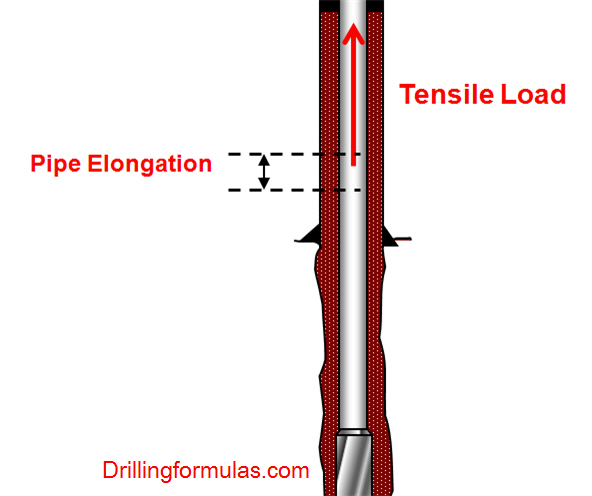The formula to determine pipe elongation is listed below;

Oilfield unit

e = (L x T) ÷ (735,000 x W)

Where;

e = elongation, inch

L = length of drill string, ft

T = tensile load, lb

W = drill string weight, lb/ft

Metric (SI) unit

e = (L x T) ÷ (26.7 x W)

Where;

e = elongation, mm

L = length of drill string, metres

T = tensile load, kN

W = drill string weight, kg/m

Note: The equations are taken from API RP 7G 16th Edition, August 1998.

## Directional Drilling Handout from Texas AM

I would like to share very useful Power Point slide in the topic of directional drilling which is belong to Texas A&M university, USA.

Directional Drilling

When is it used?

Type I Wells (build and hold)

Type II Wells (build, hold and drop)

Type III Wells (build)

Directional Well Planning & Design

Survey Calculation Methods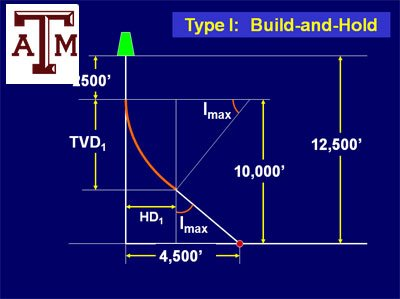Survey Calculation Methods –

Balanced Tangential

Minimum Curvature

Kicking Off from Vertical

Controlling Hole Angle (Inclination)Directional Drilling  Continued

Tool-Face Angle

Ouija Board

Dogleg Severity

Reverse Torque of Mud MotorThe Wellbore Trajectory Control –

Bent Motor and Bent Sub

Examples

Directional Drilling Measurements

Single Shot and Multishot

Magnetic and Gyro

Steering Tools

MWD tools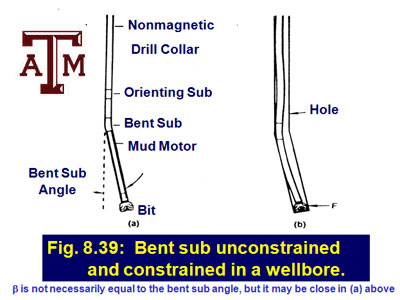## Pressure Drop Across a Bit

Pressure across a bit occurs when the drilling mud passing through the jet nozzles. This pressure drop is important for drilling hydraulic optimization (maximum hydraulic horse power or impact force) and hole cleaning. The pressure drop at the nozzle area is a function of total flow area of the bit, flow rate and mud density.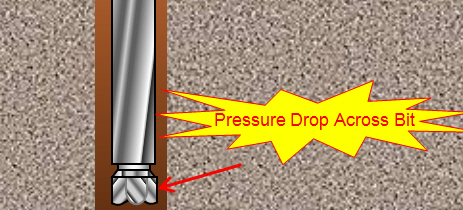Pressure drop across the bit can be determined by the following equation: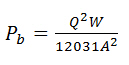Where:

Pb = pressure drop across a bit, psi

Q = flow rate, gpm

W = mud weight, ppg

A = total flow area, square inch